# Equivalent expressions with distribution and combining like terms

##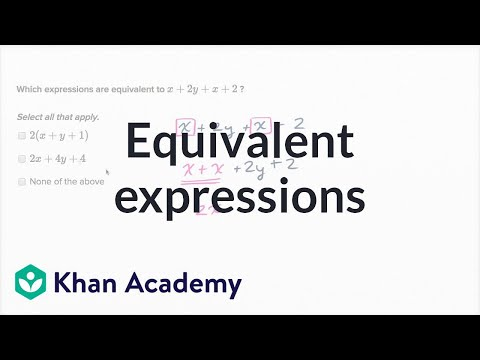By Khan Academy

Equivalent algebraic expressions exercise# Negative number addition and subtraction: equivalent expressions

##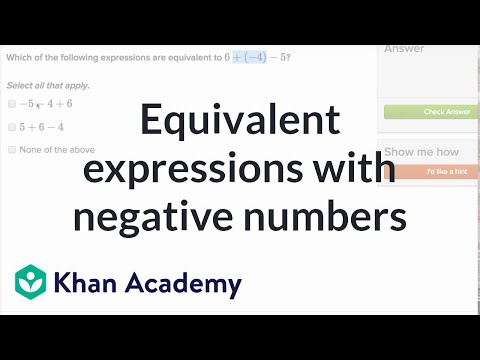By Khan Academy

Equivalent expressions with negative numbers# Equivalent expressions with negative numbers and the distributive property

##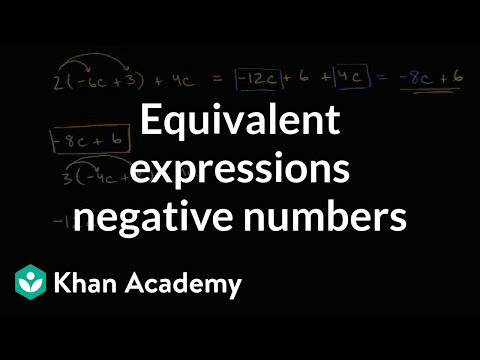By Khan Academy

Learn how to identify equivalent expressions using your knowledge of the distributive property and negative numbers.# Negative number addition and subtraction: equivalent expressions

##By Khan Academy

Equivalent expressions with negative numbers# Negative number addition and subtraction: equivalent expressions

##By Khan Academy

Equivalent expressions with negative numbers# Negative number addition and subtraction: equivalent expressions

##By Khan Academy

Equivalent expressions with negative numbers# Equivalent expressions with distribution and combining like terms

##By Khan Academy

Equivalent algebraic expressions exercise# Negative number addition and subtraction: equivalent expressions

##By Khan Academy

Equivalent expressions with negative numbers# Grade 6 Math - Writing Equivalent Expressions

##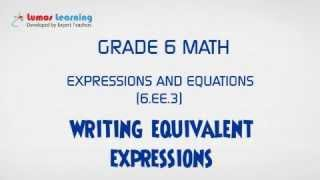By Lumos Learning

Using the Lumos Study Programs, parents and educators can reinforce the classroom learning experience for children and help them succeed at school and on the standardized tests. Lumos books, dvd, eLearning and tutoring are used by leading schools, libraries and thousands of parents to supplement classroom learning and improve student achievement in the standardized tests.# Grade 6 Math - Writing Equivalent Expressions 1

##By Lumos Learning

Using the Lumos Study Programs, parents and educators can reinforce the classroom learning experience for children and help them succeed at school and on the standardized tests. Lumos books, dvd, eLearning and tutoring are used by leading schools, libraries and thousands of parents to supplement classroom learning and improve student achievement in the standardized tests.

PARENTS please visit LumosTestPrep.com to learn more.
EDUCATORS please visit LumosLearning.com to learn more# [6.EE.4-1.0] Identify Equivalent Expressions - Common Core Standard

##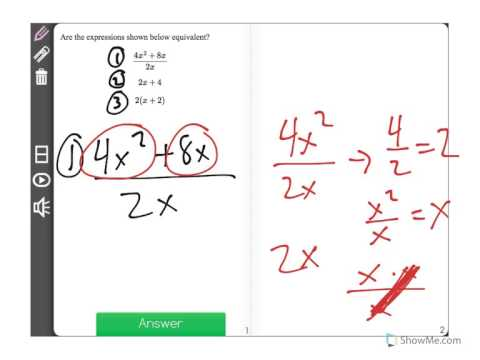By Freckle by Renaissance

Identify when two expressions are equivalent (i.e., when the two expressions name the same number regardless of which value is substituted into them). For example, the expressions y + y + y and 3y are equivalent because they name the same number regardless of which number y stands for. Front Row is a free, adaptive, Common Core aligned math program for teachers and students in kindergarten through eighth grade. Front Row allows students to practice math at their own pace - learning advanced concepts when they're ready and receiving remediation when they struggle. Front Row provides teachers with access to a detailed data dashboard and weekly email reports that show which standards are causing students difficulty, what small groups can be formed for interventions, and how their students are progressing in math.# [3.NF.3c-1.0] Whole Numbers as Fractions - Common Core Standard

##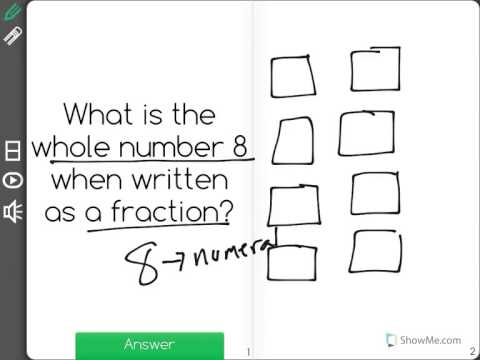By Freckle education

Explain equivalence of fractions in special cases. Express whole numbers as fractions, and recognize fractions that are equivalent to whole numbers.# Fractions to whole numbers (3.NF.3C)

##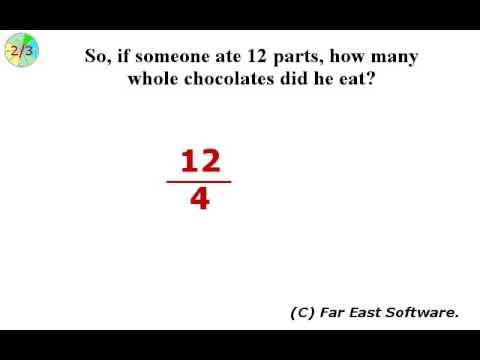By Far East Software

Express whole numbers as fractions, and recognize fractions that are equivalent to whole numbers.# Fractions to whole numbers (3.NF.3C)

##By Far East Software

Express whole numbers as fractions, and recognize fractions that are equivalent to whole numbers.# Adding Fractional Parts of 10 and 100

##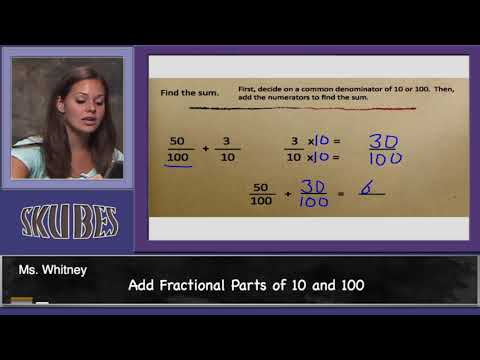By Skubes ed

Express a fraction with denominator 10 as an equivalent fraction with denominator 100# Equivalent forms of exponential expressions

##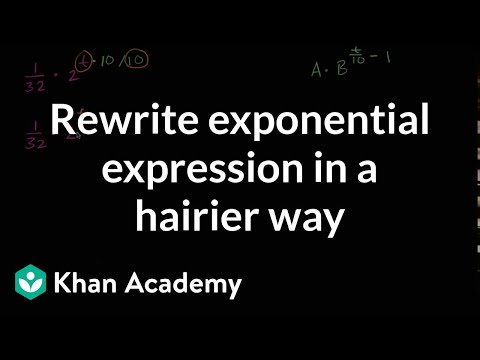By Khan Academy

Sal rewrites (1/32)*2^t as 32*1024^(t/10-1).# Equivalent forms of exponential expressions

##By Khan Academy

Sal rewrites (1/32)*2^t as 32*1024^(t/10-1).# Equivalent forms of exponential expressions

##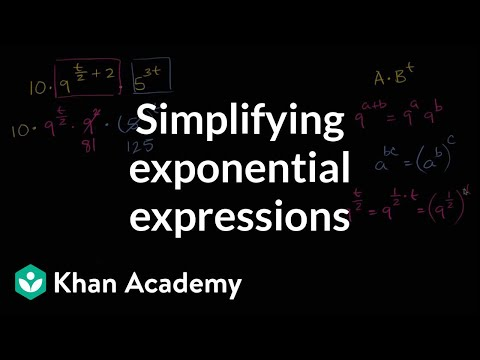By Khan Academy

Sal simplifies the expression 10*9^(0.5t+2)*5^(3t) as 810*375^t.# Equivalent forms of exponential expressions

##By Khan Academy

Sal rewrites (1/32)*2^t as 32*1024^(t/10-1).# Equivalent forms of exponential expressions

##By Khan Academy

Sal simplifies the expression 10*9^(0.5t+2)*5^(3t) as 810*375^t.